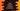# Java program to check if a number is a tech number or not## Java program to check if a number is a tech number or not:

In this post, we will learn how to check if a number is a tech number or not. This program will take one number as input from the user and print one message if it is a tech number or not.

### What is a tech number:

A number is called a tech number if:

• It can be divided into exactly two equal parts
• Divide the number into the parts and find the sum of these parts.
• Find the square of the sum
• Check if it is equal to the original number or not. If yes, it is a tech number.

### Example of tech number:

For example, 9801 is a tech number.

• Divide it in two parts: 98 and 01.
• Add the parts: 98 + 01 = 99
• Find the square of 99: 9801

It is equal to the original number. So, 9801 is a tech number.

### Algorithm to check if a number is tech number or not:

We will use the following algorithm to check for a tech number:

• Get the number from the user.
• Divide the number in two parts. We will use modulo % operator to divide the number by multiple of 10 to get the remainder and
• Add these parts to find the sum.
• Find the square of the sum.
• Compare this value with the original number. If both are equal, this is a tech number.

### Java program:

``````import java.util.Scanner;

public class Main {
public static void main(String[] args) {
int number, temp, totalDigits = 0;
Scanner sc = new Scanner(System.in);

System.out.println("Enter a number: ");
number = sc.nextInt();

temp = number;

while (temp > 0) {
totalDigits++;
temp = temp / 10;
}

if (totalDigits % 2 == 0) {
int div = (int) Math.pow(10, totalDigits / 2);
int firstHalfOfNumber = number / div;

int secondHalfOfNumber = number % div;

int sumSquare = (firstHalfOfNumber + secondHalfOfNumber) * (firstHalfOfNumber + secondHalfOfNumber);

if (sumSquare == number) {
System.out.println(number + " is a tech number");
} else {
System.out.println(number + " is not a tech number");
}
} else {
System.out.println(number + " is not a tech number");
}

}
}``````

Here,

• number is to hold the user input number, temp is a temporary variable and totalDigits is to hold the total number of digits of the number.
• sc is a Scanner object used to read user inputs.
• It is asking the user to enter a number. It reads that number and stores that in the number variable.
• It stores the value in the temporary variable and uses a while loop to find the length of the number or total number of digits of the number. totalDigits variable is used to hold that value.
• The if block checks if the total number of digits is even or not.
• If it is even, we are dividing the number in two parts and finding the square of the sum of these parts.
• If the sum of square is equal to the number, it is printed as a tech number. Else, it is not.

### Sample output:

If you run this program, it will print output as like below:

``````Enter a number:
2025
2025 is a tech number

Enter a number:
2015
2015 is not a tech number``````

## Method 2: By converting the integer to a string:

We can also solve this by converting the integer to a string. We can convert the integer to a string and get the first and second half of the string and convert them to integers to solve it in a same way.

``````import java.util.Scanner;

public class Main {
public static void main(String[] args) {
int number;
Scanner sc = new Scanner(System.in);

System.out.println("Enter a number: ");
number = sc.nextInt();

String strNum = String.valueOf(number);

if (strNum.length() % 2 == 0) {
int firstHalfOfNumber = Integer.parseInt(strNum.substring(0, strNum.length() / 2));

int secondHalfOfNumber = Integer.parseInt(strNum.substring(strNum.length() / 2));

int sumSquare = (firstHalfOfNumber + secondHalfOfNumber) * (firstHalfOfNumber + secondHalfOfNumber);

if (sumSquare == number) {
System.out.println(number + " is a tech number");
} else {
System.out.println(number + " is not a tech number");
}
} else {
System.out.println(number + " is not a tech number");
}

}
}``````

Here,

• strNum is the string value of the number.
• We are parsing the first half of the string to an integer by using Integer.parseInt and similarly we are parsing the second half of the string.
• The other parts are similar to the above program. sumSquare is the square value of the sum of the first and these second half.

If you run this program, it will give similar output.

``````Enter a number:
20153
20153 is not a tech number``````